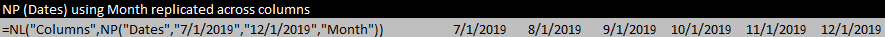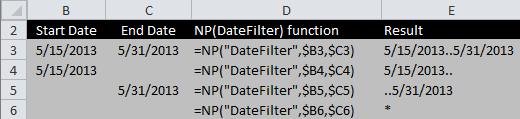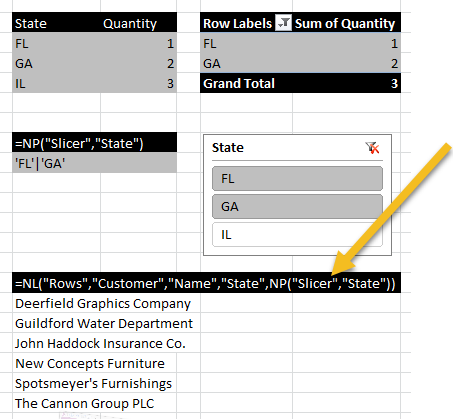Community
Training
Submit a Request
Become a Jet Insider
Give Feedback

# NP Function Reference

Related Articles...

## =NP (What, Arg1, Arg2, ...,Arg22)

Purpose:  Performs utility oprations.

Sometimes you need to do certain utility tasks such as formatting a date range, evaluating a function/formula, or doing array calculations.

The Jet Utility function, NP, will do these tasks for you.  (examples)

## Function Reference

### What

• "Codeunit" (Dynamics NAV only)

Evaluates and returns the value returned by the Dynamics NAV code unit function.

If connecting to the database through NAV Web Services, any of NAV's Authentication Methods may be used.  Other connection types (SQL Direct, C/Front, JRDS) require Windows Authentication.

• "Companies"

Returns a list of the companies associated with a data source.

Arg1 is a company filter such as A* to return all companies that start with the letter A.  Leaving Arg1 blank will return all companies.

Arg2 is the data source.  Leaving Arg2 blank will return companies from the current data source.

Note that you should reference the result of this function in the table argument of an NL replicator function [ NL("Rows"), NL("Columns"), or NL("Sheets") ] to actually list them out in Excel.
• "Datasources"

Returns an array containing the current user's Jet data sources.

• "DateFilter"

Calculates a date filter using the start date and end date specified in the Arg1 and Arg2 parameters.  Also see Date Filters

• "Dates"

Returns a string that can be used to generate dates using a Replicator, where:

• Arg1 is the start date
• Arg2 is the end date
• Arg3 can be used to specify a period type of Day, Week, Month, Quarter, or Year.  Default is Day.
• Arg4 can be set to "True" in order to return the end of each period.  Default is "False".
• "Difference"

Returns a Jet-specific list containing the difference between the two arrays specified in the Arg1 and Arg2 parameters.  Also see Array Calculations

Note that if NP("Difference") is by itself in a cell, it will only return the first value from the array. You must put it inside an NL("Rows") in order to correctly return all the data.
• "Eval"

Evaluates the formula in the Arg1 parameter. The formula must be enclosed in quotes and will be evaluated when the report refreshes.

• "Format"

FOR BACKWARD COMPATIBILITY - DO NOT USE or USE EXCEL'S TEXT() FUNCTION

Formats an expression with a specific Excel formatting string. Arg1 is the expression to format such as a date or cell reference, and Arg2 is the Excel formatting string such as "YYYY/MM/DD" for a date formatted with a 4-digit year then a 2-digit month and 2-digit day.

• "Formula"

NOTE: ADD "UseLocalFormulas=","True" to your NL("Table" function if your Excel formulas are in a language other than English.

Evaluates the Excel formula contained within Arg1.

• "Integers"

Returns a string that can be used to generate integers using a Replicator, where Arg1 is the start number and Arg2 is the end number.

• "Intersect"

Returns a Jet-specific list containing the intersection of the two arrays specified in the Arg1 and Arg2 parameters.  Also see Array Calculations

Note that in versions of Jet Essentials 2015 and earlier, if NP("Intersect") is by itself in a cell, it will only return the first value from the array.  For those versions, you must put it inside an NL("Rows") in order to correctly return all the data.
• "Join"

Joins the elements of the array specified in Arg1 together into a single string separated by the contents of Arg2.

• "Slicer"

Converts an Excel Slicer to a filter that can be used in Jet functions.  In Report Mode, the NP(Slicer) function refreshes every time the Excel Slicer changes. The NP(Slicer) function can be embedded in other Jet functions or can be placed in its own cell allowing multiple functions to reference it as a filter.

The Excel Slicer referenced by the NP(Slicer) function must be associated with at least one pivot table in order for the NP(Slicer) function to update properly.

• "Split"

In versions of Jet Essentials 2015 Update 1 and higher, this function splits the string in Arg1 into a Jet-specific list.

In earlier versions, this function splits the string in Arg1 into an array of values (instead of a Jet-specific list). The splitting is delimited by the contents of Arg2.

If NP("Split") is by itself in a cell, it will only return the first value from the array. You must put it inside an NL("Rows") in order to correctly return all the data.
• "Union"

Returns a Jet-specific list containing the Union of the two arrays specified in the Arg1 and Arg2 parameters.  Also see Array Calculations

In versions of Jet Essentials 2015 and earlier, if NP("Union") is by itself in a cell, it will only return the first value from the array.  For those versions, you must put it inside an NL("Rows") in order to correctly return all the data.

## Examples of the NP function

#### EVAL - The following NP evaluates the formula in cell of D5 from a worksheet called Options.

`=NP("Eval","=Options!\$D\$5")`

Excel handles cross-sheet references slower than on-sheet.  While recent versions of Jet Reports include optimization to correct for this, earlier versions of Jet Reports would perform better if off-sheet references were copied to the current worksheet.

#### INTEGERS- This NP(Integers) function will create rows with the numbers 1 through 10.

`=NL("Rows",NP("Integers",1,10))`

#### INTERSECT- The following example takes the intersection of two arrays.

`=NP("Intersect",{"1","2","3"},{"2","3","4"})`

This function will return {2,3}.

See also: our Array Calculations article discussing functions NL("Filter"), NP("Join"), NP("Union"), and NP("Split")

#### JOIN- The following NP(Join) joins the strings from an array and creates the result " 100|200|300|400" for potential use in another function.

`=NP("Join",{"100","200","300","400"},"|")`

#### SPLIT - The following NP(Split) splits up the string "this|is|an|array" and creates a Jet-specific lists containing this, is, my, list

`=NP("Split", "this|is|my|list", "|")`

#### COMPANIES- The following NP(Companies) function lists all the companies for the current data source in rows.

`=NL("Rows",NP("Companies"))`

#### DATES- The use of NP(Dates) to create a set of column headers for a report. (Dates can also be placed in reverse order by putting the later date in first)

`=NL("Columns",NP("Dates","7/1/2019","12/1/2019","Month"))`

#### DATEFILTER - Results of using the NP(DateFilter) function, which can then be nested in other functions.

``

#### Format a date range from 1/1/22 to 12/31/22

`=NP("DateFilter,"1/1/22","12/31/22")`

#### DATASOURCES - This NP(DataSources) function will return a list of the data sources in use on the machine it is run on.

`=NL("Rows",NP("Datasources"))`

#### FORMULA - Used in conjunction with the NL(Table) function to define a calculated column in the table definition.   For example: To determine available credit for a customer; if cell E6 contains the credit limit, and cell F6 contains the open credit, then

`=NP("Formula","=E6-F6")`

would be put in the field list of the NL(Table) definition

#### SLICER - The Slicer function works in conjunction with pivot tables and dashboards to provide information for filters when refreshing reports.

``